## Selina Concise Mathematics Class 10 ICSE Solutions Chapter 8 Remainder and Factor Theorems Ex 8A

These Solutions are part of Selina Concise Mathematics Class 10 ICSE Solutions. Here we have given Selina Concise Mathematics Class 10 ICSE Solutions Chapter 8 Remainder and Factor Theorems Ex 8A.

Other Exercises

Question 1.
Find in each case, the remainder when :
(i) x4 – 3x2 + 2x + 1 is divided by x – 1.
(ii) x3 + 3x2 – 12x + 4 is divided by x – 2.
(iii) x4 + 1 is divided by x + 1.
(iv) 4x3 – 3x2 + 2x – 4 is divided by 2x + 1.
(v) 4x3 + 4x2 – 21x + 16 is divided by 2x – 3.
(vi) 2x3 + 9x2 – x – 15 is divided by 2x + 3.
Solution:Question 2.
Show that:
(i) x – 2 is a factor of 5x2 + 15x – 50.
(ii) 3x + 2 is a factor of 3x2 – x – 2.
(iii) x + 1 is a factor of x3 + 3x2 + 3x + 1.
Solution: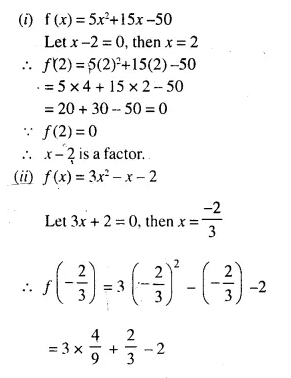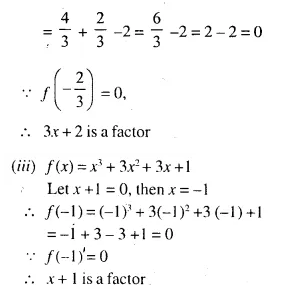Question 3.
Use the Remainder Theorem to find which of the following is a factor of 2x3 + 3x2 – 5x – 6.
(i) x + 1
(ii) 2x – 1
(iii) x + 2
(iv) 3x – 2
(v) 2x – 3.
Solution:
(i) f(x) = 2×3 + 3×2 – 5x – 6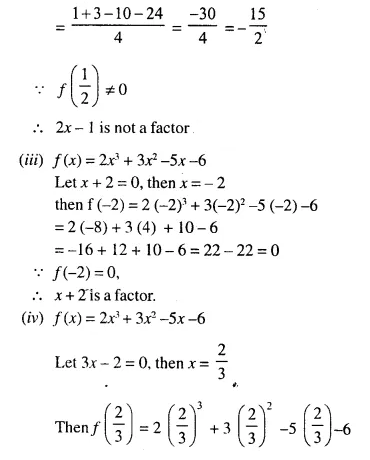Question 4.
(i) If 2x + 1 is a factor of 2x2 + ax – 3, find the value of a.
(ii) Find the value of k, if 3x – 4 is a factor of expression 3x2 + 2x – k.
Solution: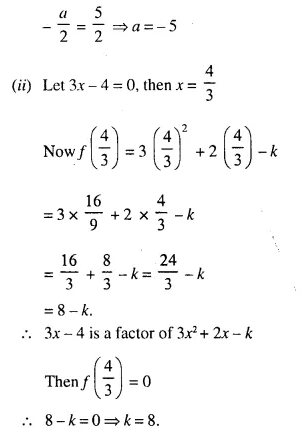Question 5.
Find the values of constants a and b when x – 2 and x + 3 both are the factors of expression, x3 + ax2 + bx – 12.
Solution: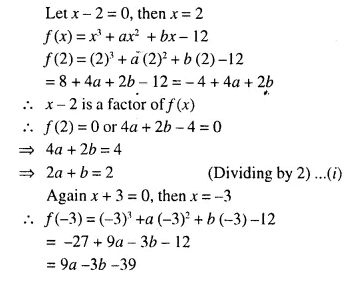(x + 3) is a factor of f(x)
f(-3) = 0
9a – 3b – 39 – 0
⇒ 3a – b – 13 = 0
⇒ 3a – b = 13
Adding (i) and (ii) we get:
5a = 15 ⇒ a = 3
Substituting the value of a in (i)
2(3) + b = 2
⇒ 6 + b = 2
⇒ b = 2 – 6 = – 4
Hence a = 3, b = – 4

Question 6.
Find the value of k, if 2x + 1 is a factor of (3k + 2) x3 + (k – 1).
Solution:Question 7.
Find the value of a, if x – 2 is a factor of 2x5 – 6x4 – 2ax3 + 6ax2 + 4ax + 8.
Solution: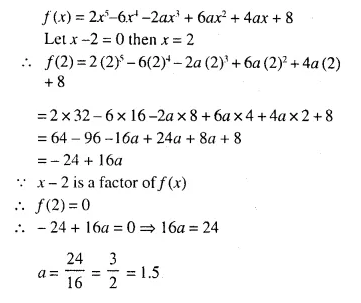Question 8.
Find the values of m and n so that x – 1 and x + 2 both are factors of x3 + (3m + 1) x2 + nx – 18.
Solution: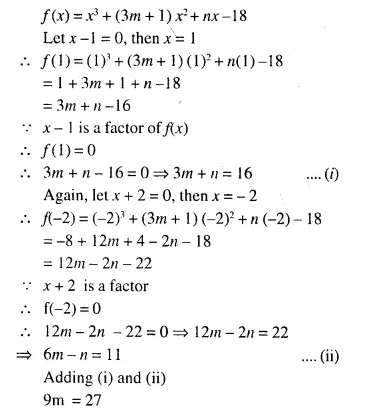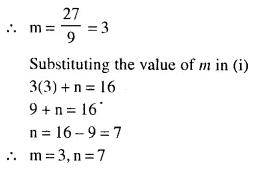Question 9.
When x3 + 2x2 – kx + 4 is divided by x – 2, the remainder is k. Find the value of constants.
Solution:
f(x) = x3 + 2x2 – kx + 4Question 10.
Find the value of a, if the division of ax3 + 9x2 + 4x – 10 by x + 3 leaves a remainder 5.
Solution: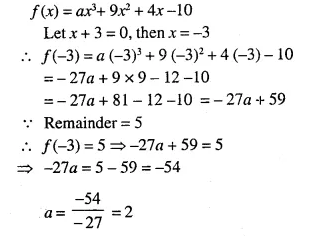Question 11.
If x3 + ax2 + bx + 6 has x – 2 as a factor and leaves a remainder 3 when divided by x – 3, find the values of a and b. 
Solution:Question 12.
The expression 2x3 + ax2 + bx – 2 leaves remainder 7 and 0 when divided by 2x – 3 and x + 2 respectively. Calculate the values of a and b.
Solution: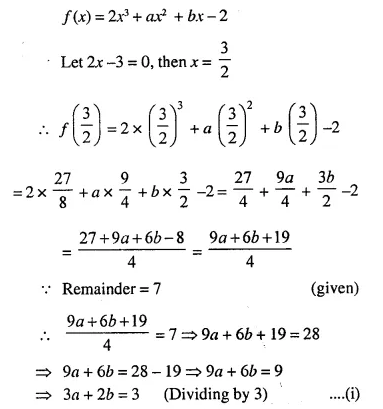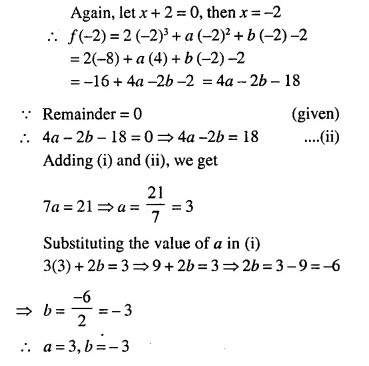Question 13.
What number should be added to 3x3 – 5x2 + 6x so that when resulting polynomial is divided by x – 3, the remainder is 8 ?
Solution: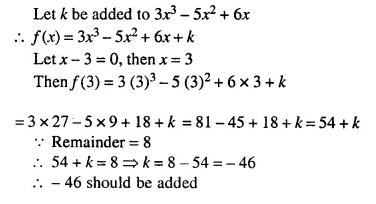Question 14.
What number should be subtracted from x3 + 3x2 – 8x + 14 so that on dividing it by x – 2, the remainder is 10.
Solution: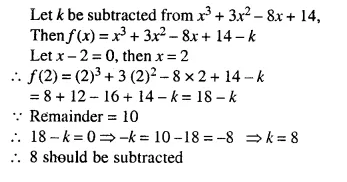Question 15.
The polynomials 2x3 – 7x2 + ax – 6 and x3 – 8x2 + (2a + 1) x – 16 leave the same remainder when divided by x – 2. Find the value of ‘a’.
Solution:Question 16.
If (x – 2) is a factor of the expression 7x3 + ax2 + bx – 14 and when the expression is divided by (x – 3), it leaves a remainder 52, find the values of a and b.
Solution:Question 17.
Find ‘a’ if the two polynomials ax3 + 3x2 – 9 and 2x3 + 4x + a, leave the same remainder when divided by x + 3. (2015)
Solution: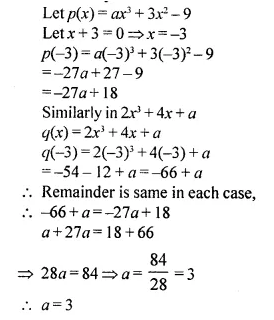Hope given Selina Concise Mathematics Class 10 ICSE Solutions Chapter 8 Remainder and Factor Theorems Ex 8A are helpful to complete your math homework.

If you have any doubts, please comment below. Learn Insta try to provide online math tutoring for you.